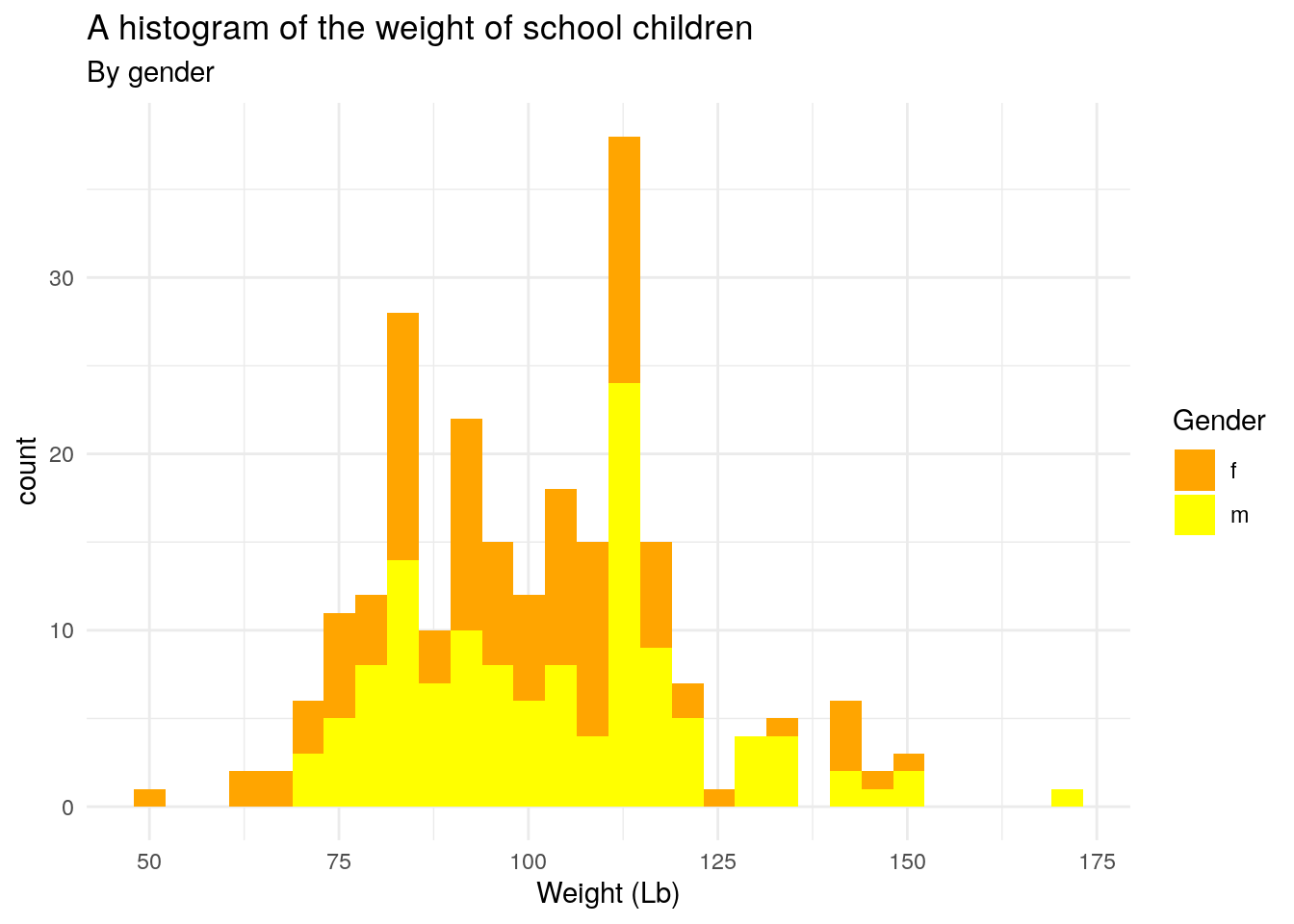# Chapter 1 Exercise

``install.packages("tidyverse", repos = "http://cran.us.r-project.org")``
``````## Installing package into '/opt/rstudio-connect/mnt/report/packrat/lib/x86_64-pc-linux-gnu/3.6.2'
## (as 'lib' is unspecified)``````
``library(tidyverse)``
``## ── Attaching packages ─────────────────────────────────────── tidyverse 1.3.0 ──``
``````## ✔ ggplot2 3.3.3     ✔ purrr   0.3.4
## ✔ tibble  3.0.6     ✔ dplyr   1.0.4
## ✔ tidyr   1.1.3     ✔ stringr 1.4.0
## ✔ readr   1.4.0     ✔ forcats 0.5.1``````
``## Warning: package 'ggplot2' was built under R version 3.6.3``
``````## ── Conflicts ────────────────────────────────────────── tidyverse_conflicts() ──
``install.packages("gcookbook", repos = "http://cran.us.r-project.org")``
``````## Installing package into '/opt/rstudio-connect/mnt/report/packrat/lib/x86_64-pc-linux-gnu/3.6.2'
## (as 'lib' is unspecified)``````
``library(gcookbook)``

Exercise 3-1

``head(heightweight)``
``````##   sex ageYear ageMonth heightIn weightLb
## 1   f   11.92      143     56.3     85.0
## 2   f   12.92      155     62.3    105.0
## 3   f   12.75      153     63.3    108.0
## 4   f   13.42      161     59.0     92.0
## 5   f   15.92      191     62.5    112.5
## 6   f   14.25      171     62.5    112.0``````
``````#Exercise 3-1-a
ggplot(heightweight, aes(x = ageYear, y = heightIn, colour = sex, size = weightLb)) + geom_point()``````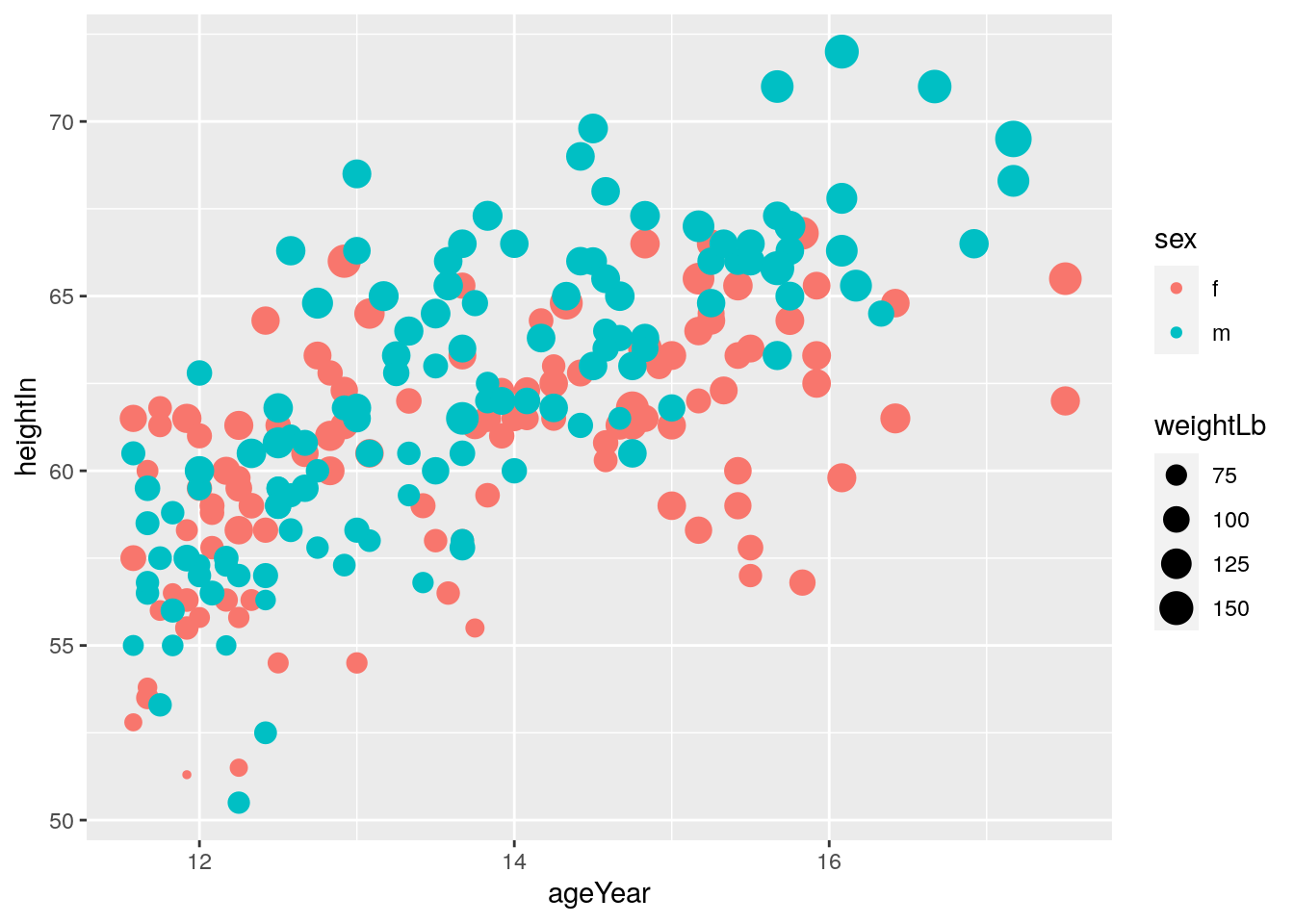``````#Exercise 3-1-b
ggplot(heightweight, aes(x = ageYear, y = heightIn, colour = sex, size = weightLb)) + geom_point(alpha = 0.3)``````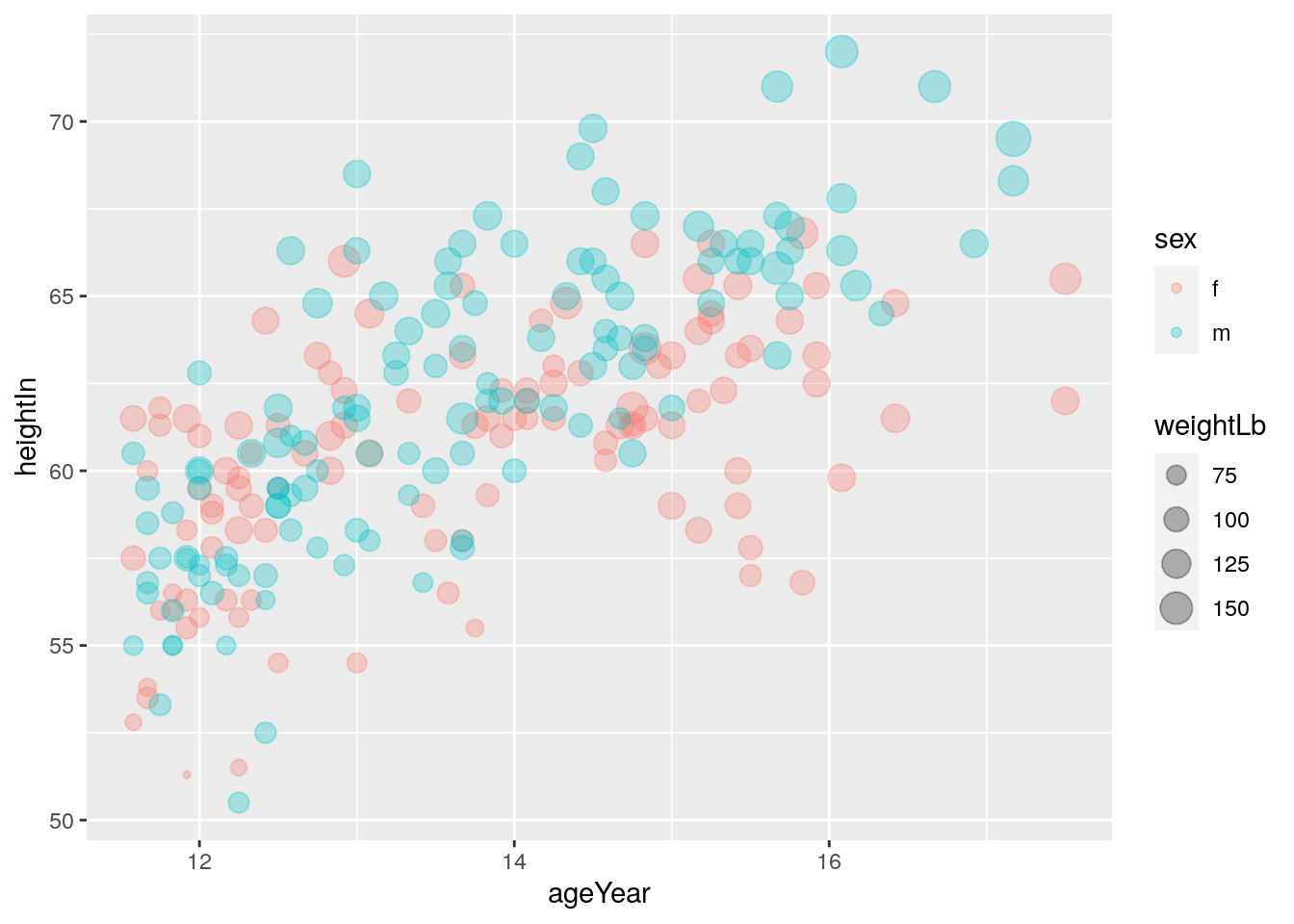``````#Exercise 3-1-c
ggplot(heightweight, aes(x = ageYear, y = heightIn, color = sex, size = weightLb)) + geom_point(alpha = 0.3) + labs(title = "Height and weight of school children", subtitle = "Height vs Weight", x = "Age (year)", y = "Height (inches)", color = "Gender", size = "Weight (Lb)", caption = "Source: heightweight")``````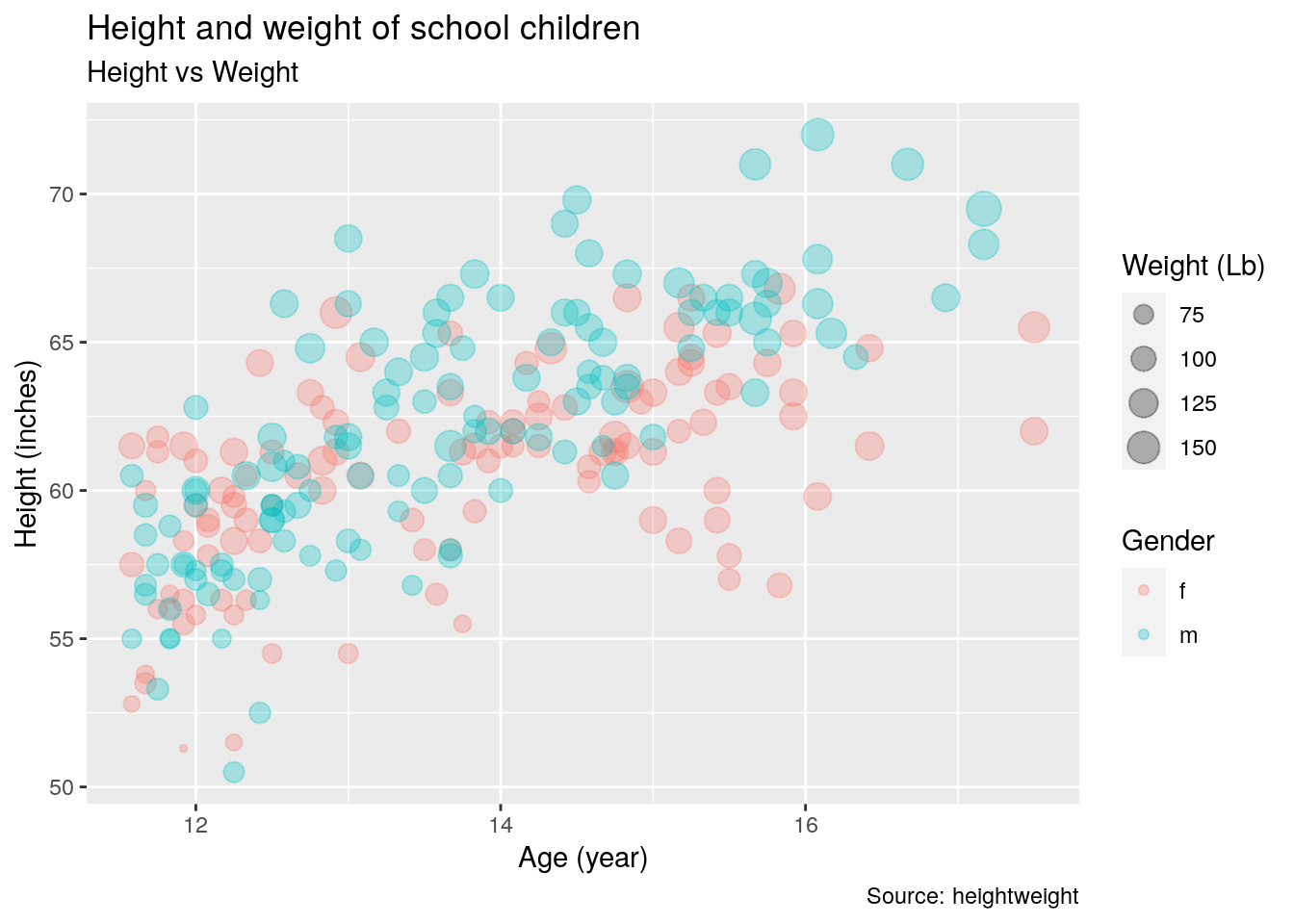``````#Exercise 3-1-d
ggplot(heightweight, aes(x = ageYear, y = heightIn, color = sex, size = weightLb)) + geom_point(alpha = 0.3) + labs(title = "Height and weight of school children", subtitle = "Height vs Weight", x = "Age (year)", y = "Height (inches)", color = "Gender", size = "Weight (Lb)") + theme_classic()``````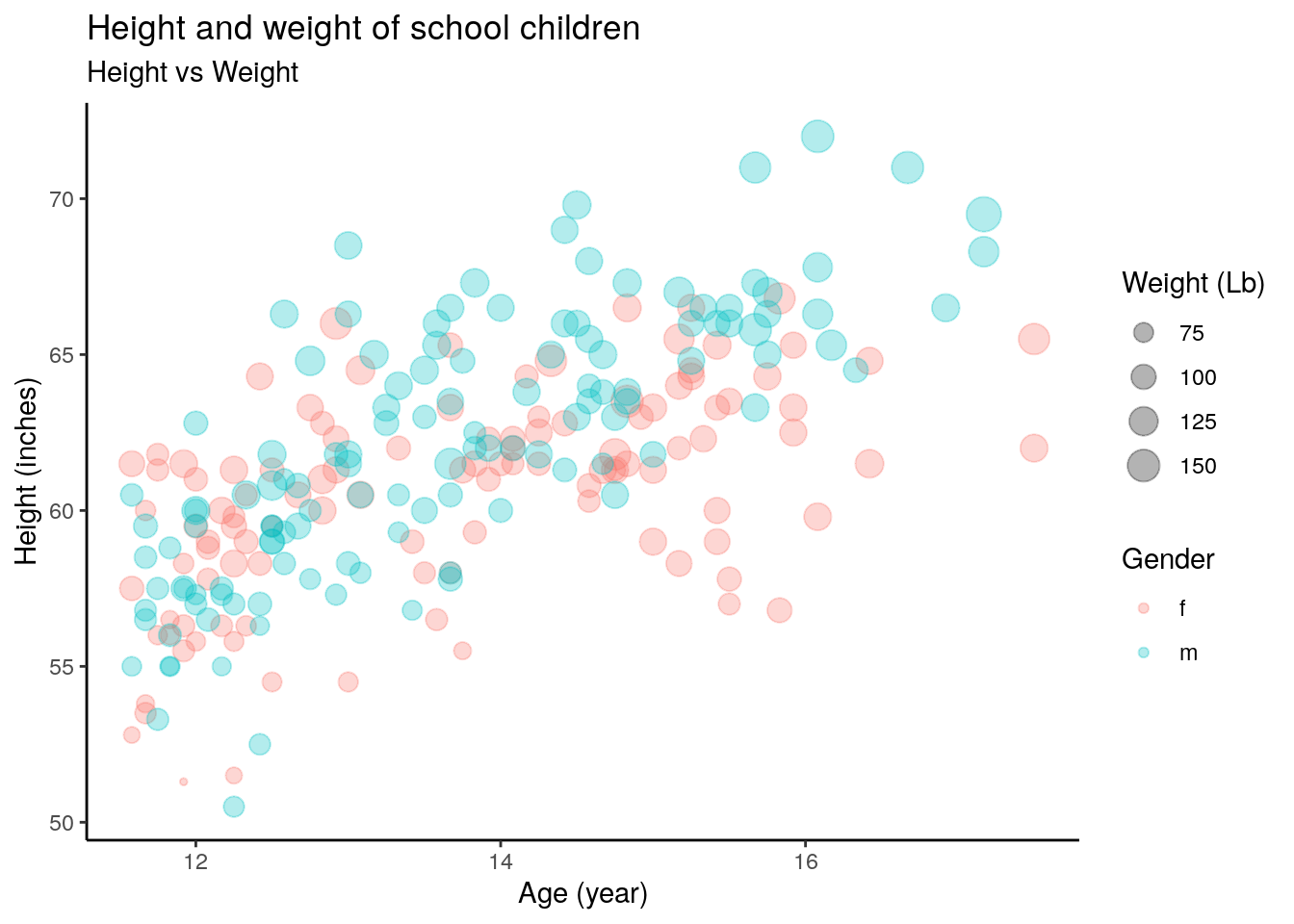Exercise 3-2

``head(heightweight)``
``````##   sex ageYear ageMonth heightIn weightLb
## 1   f   11.92      143     56.3     85.0
## 2   f   12.92      155     62.3    105.0
## 3   f   12.75      153     63.3    108.0
## 4   f   13.42      161     59.0     92.0
## 5   f   15.92      191     62.5    112.5
## 6   f   14.25      171     62.5    112.0``````
``````#Exercise 3-2-a
ggplot(heightweight, aes(x = heightIn)) + geom_histogram()``````
``## `stat_bin()` using `bins = 30`. Pick better value with `binwidth`.``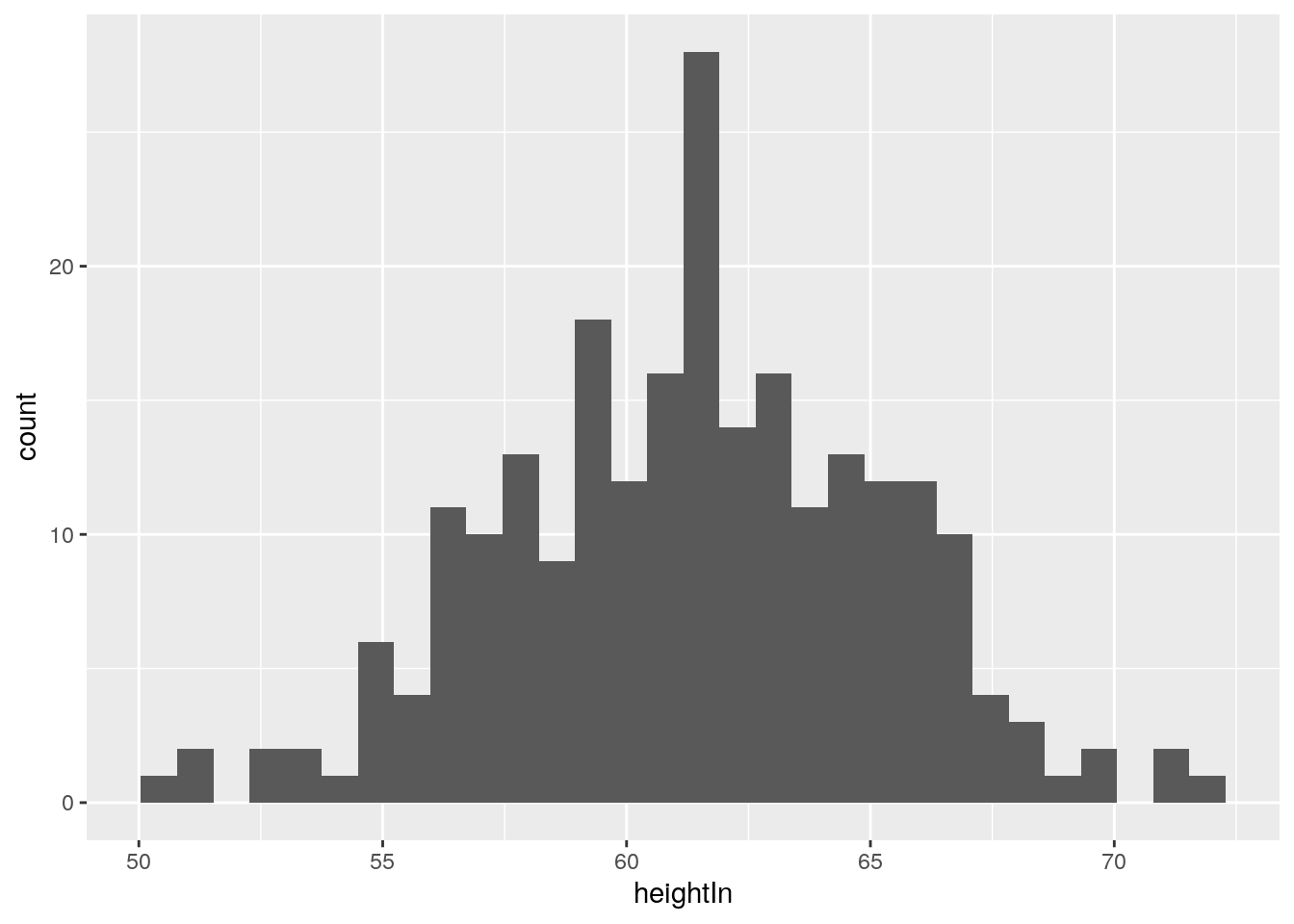``````#Exercise 3-2-b
ggplot(heightweight, aes(x = weightLb, fill = sex)) + geom_histogram() + labs(title = "Height and weight of school children", subtitle = "by Gender", x = "Age (year)", color = "Gender", size = "Weight (Lb)", caption = "Source: heightweight") + theme_classic()``````
``## `stat_bin()` using `bins = 30`. Pick better value with `binwidth`.``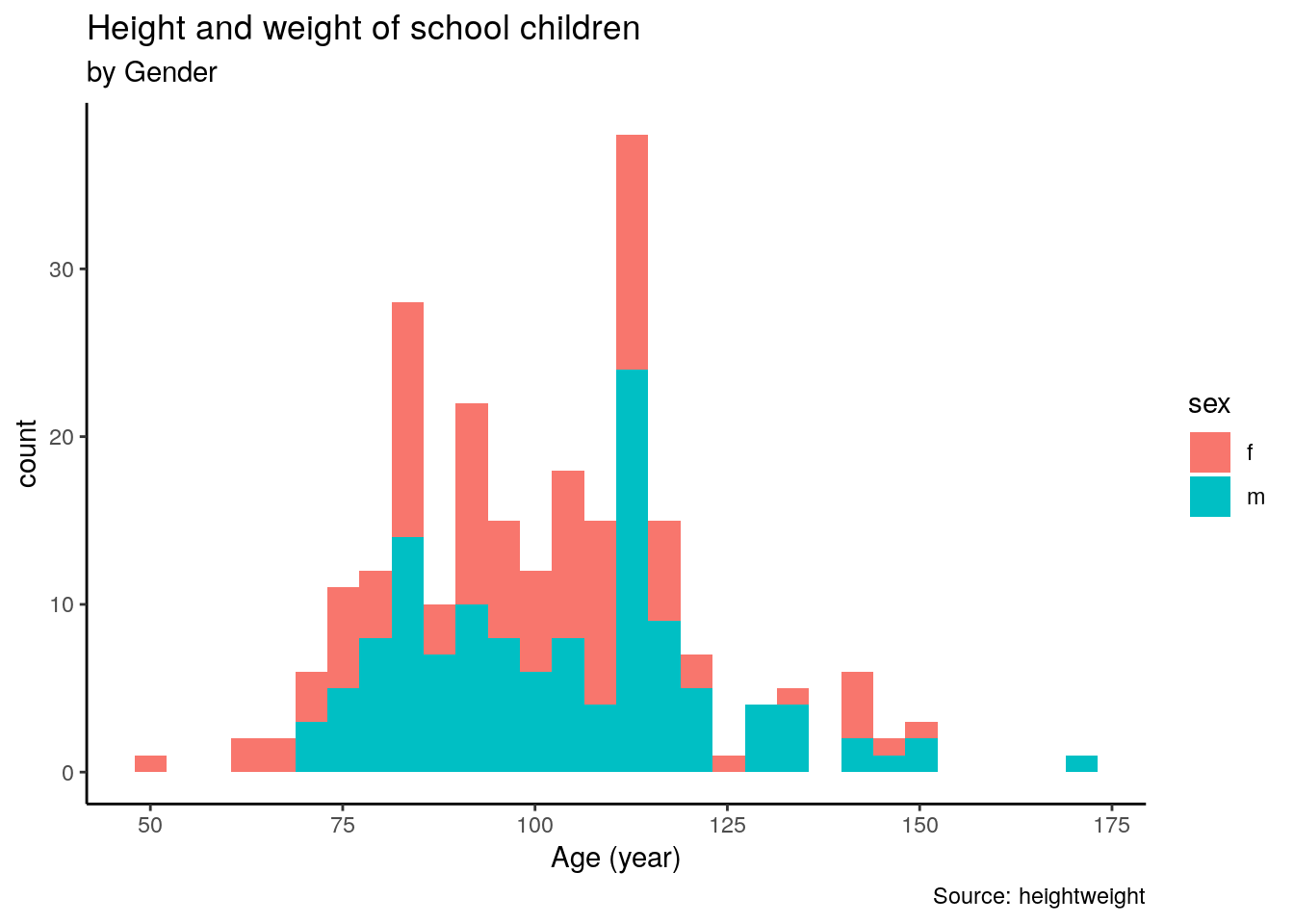``````#Exercise 3-2-c
ggplot(heightweight, aes(x = weightLb, fill = sex)) + scale_colour_manual(values = c("orange", "yellow")) + scale_fill_manual(values = c("orange", "yellow")) + geom_histogram()``````
``## `stat_bin()` using `bins = 30`. Pick better value with `binwidth`.``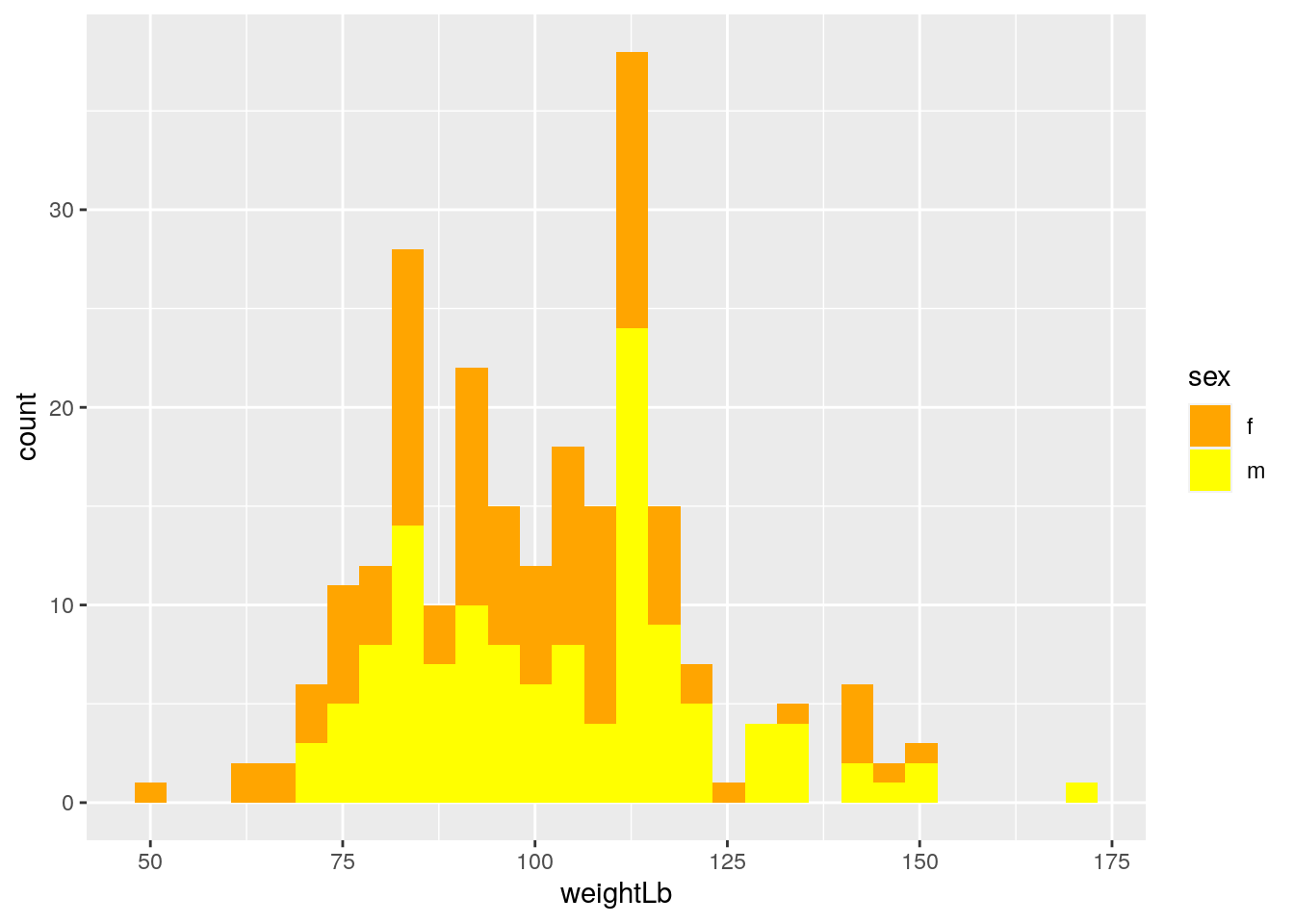``````#Exercise 3-2-d
ggplot(heightweight, aes(x = weightLb, fill = sex)) + scale_colour_manual(values = c("orange", "yellow")) + scale_fill_manual(values = c("orange", "yellow")) + geom_histogram() + labs(title = "A histogram of the weight of school children", subtitle = "By gender", x = "Weight (Lb)", fill = "Gender") + theme_minimal()``````
``## `stat_bin()` using `bins = 30`. Pick better value with `binwidth`.``